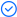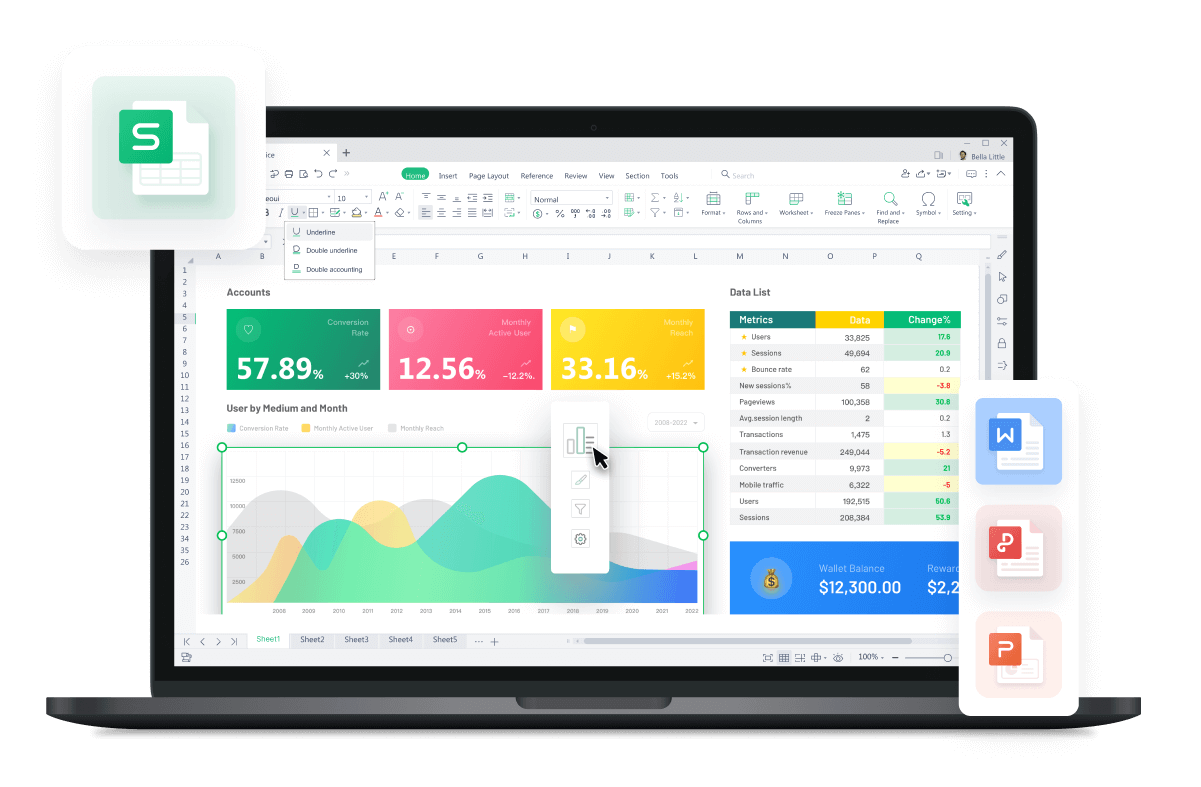WPS Office

Free All-in-One Office Suite with PDF EditorRead, edit, and convert PDFs with the powerful PDF toolkit.Microsoft-like interface, easy to use.

Windows • MacOS • Linux • iOS • Android# How to use sumif in excel (Easy Steps)

August 1, 2022
1.5K Views

It is time to learn how to use sumif in Excel if you want to sum multiple cells in the working file. As an employee who uses Excel constantly, you should familiarize yourself with the SUMIF function knowing that you may need it at some point. With this function, you will add dates, texts, wildcards, and other things; you must know how to use it.

It is good that you look for guides on how to use sumif in Excel to apply the formula correctly. You can also look for examples where the formula is applied and thus enter the context of how it works.

How to use SUMIF in Excel in a simple way?

To know how to use sumif in excel 2019, you could resort to the simplest method that promises to make you understand the formula in a matter of minutes. It is good to know that this formula is available in Excel 2007, so you will not have any problem locating it in your version. The base of the formula is SUMIF (range, criteria, [sum_range]) where:

The range would correspond to the number of cells that seek to be evaluated with SUMIF.The criteria are the conditions to be fulfilled in the formula, including text or numerical factors.Sum-range is the range that will add if the rule is fulfilled. It is a point within the formula that could be functional.

Now that you have the basics of the formula, you should know how to use sumif in excel Mac:

1. Open an Excel document where it is required to use the SUMIF function. In the example, a spreadsheet containing various data took. The objective to be fulfilled in the example is to add the sales in the north region.

2. To add these sales, you will have to use the formula =SUMIF (B2:B10,F1,C2:C10), where you can see that columns B and C are included in the calculation.

3. Now, you only have to select the cell where the result will appear and click on the enter key to make it appear.

How to use SUMIF in Excel?

You can also learn how to use sumif in excel 2016 by following some examples:

EXAMPLE 1: Add numbers greater than or less than a specified value.

1. In the example, you see three sales where the items appear, item sales, and ships in, specifying the number of days. If you want a shipping calculation and the days, it will take to deliver those products. It would be best if you used the SUMIF function.

2. To execute the formula, you must indicate the range of cells that, in this case, would be from C2 to C10. Also, in the formula, you must place the range of cells B2 to B10 if you want to have the total sale budget. In this way, the formula would be like this =SUMIF(C2:C10, >3, B2:B10)

3. In case you want to do the calculation in a different cell, like F1, for example, you should create a formula like this: =SUMIF(C2:C10, >&F1, B2:B10)

4. On the other hand, you can sum the smallest values in the file using the formula =SUMIF(C2:C10,

EXAMPLE 2: to add numbers with the parameter equal to.

Another way you can find out how to use sumif in excel Online is by trying to sum numbers with the equal to parameter. In this case, you should proceed as follows:

1. You could take the previous example where there are 3 columns, one to place the items, another to indicate the sales, and the last to show the days of product delivery. If you want to add numbers with the parameter equal to, you should use the formula =SUMIF(C2:C10, 3, B2:B10) or also =SUMIF(C2:C10, =3, B2:B10).

2. Now, if you want to give a location to the result that you will have with the formula, you will have to use these criteria: =SUMIF(C2:C10, F1, B2:B10)

To know how to use sumif in excel, you will only have to pay attention to the parameters previously explained. Although the SUMIF formulas are difficult to apply in the Excel file, you may be able to master them with some practice. You have to motivate yourself to learn more about this formula and take great advantage of it when needed.

If the information on how to use sumif in Excel was helpful, don't forget to thank the website that promoted this type of guide.

On the other hand, you should take advice on WPS Office and why it is necessary to have the software on your computer. With WPS Office, you can access Excel, Word, and PowerPoint without paying a subscription after downloading the software.